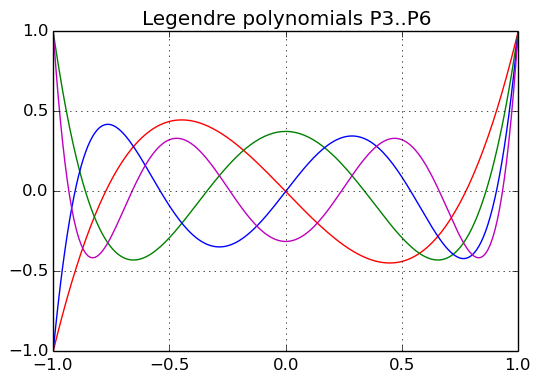## Functions and plotting

### Defining functions

The basic syntax for defining more complex functions in Julia is function fname(params) ... end. Functions will be called in the traditional f() syntax. Functions are first class objects and can be passed with, e.g., g = f.
Functions will return the last evaluated expression. The return keyword causes the function to return immediately. Multiple values are returned as tuples.

In :
# sox(x) = sin(x) / x
function sox(x)
sin(x) / x
end

Out:
sox (generic function with 1 method)

In :
sox(0.0)

Out:
NaN

In :
code_typed(sox, (Float64,))
# code_llvm(sox, (Float64,))
# code_native(sox, (Float64,))

Out:
1-element Array{Any,1}:
:($(Expr(:lambda, {:x}, {{:_var0},{{:x,Float64,0},{:_var0,Float64,18}},{}}, :(begin # In, line 3: _var0 = (GetfieldNode(Base.Math,:nan_dom_err,Any))((top(ccall))($(Expr(:call1, :(top(tuple)), "sin", GetfieldNode(Base.Math,:libm,Any)))::(ASCIIString,ASCIIString),Float64,\$(Expr(:call1, :(top(tuple)), :Float64))::(Type{Float64},),x::Float64,0)::Float64,x::Float64)::Float64
return (top(box))(Float64,(top(div_float))(_var0::Float64,x::Float64))::Float64
end::Float64))))


Function arguments are passed by reference. Changes to mutuable objects (such as arrays) will be visible to the outside.
Functions that change the internal state of (some of) their parameters should have names with a closing '!' (bang).

In :
A = {};
push!(A, 1); push!(A, [1, 2]); push!(A, "1, 2, 3")

Out:
3-element Array{Any,1}:
1
[1,2]
"1, 2, 3"


Anonymous functions are available as, e.g., x -> x * exp(x) and can be mapped onto an array of (single) parameters.

In :
map(x -> x * exp(x), [1:5])

Out:
5-element Array{Float64,1}:
2.71828
14.7781
60.2566
218.393
742.066


Julia functions can use the usual control flow elements: "forget the ':' and add an 'end' statement."
if ... elseif ... else ... end, for- and while-loops, or try ... catch for exception handling --
continue and break are statements to leave an inner loop.

In :
function agm(a, b)
while a != b
a, b = (a+b)/2.0, sqrt(a*b)
end
return a
end

Out:
agm (generic function with 1 method)

In :
agm(1.0, sqrt(2.0))  # Gauss' constant 1.19814023473559220744

Out:
1.1981402347355923


Julia functions can have optional and keyword arguments. Optional arguments are considered for method dispatch, keyword arguments not.
Optional arguments cannot be used with their name.
Keyword arguments are separated from other arguments by a semicolon.

In :
function agm(a, b, tol = 1e-10)
while abs(a - b) > tol
a, b = (a+b)/2.0, sqrt(a*b)
end
return a
end

Out:
agm (generic function with 2 methods)

In :
agm(1.0, sqrt(2.0), 1e-05)

Out:
1.1981402347938772


Julia functions can have vararg parameters ...

### Plotting functions

There are several packages for function plotting and generating statistical graphs:

• Winston -- simple function plotting
• Gaston -- uses GnuPlot
• GadFly -- implements a Grammar of Graphics (as in R's ggplot2)
• PyPlot -- uses Python's Matplotlib
In :
using PyPlot
pyplot = PyPlot.plot

INFO: Loading help data...


Out:
plot (generic function with 1 method)

In :
x = linspace(-1, 1, 201);

figure(figsize=(6.0, 4.0));
pyplot(x, map(x->(5x^3-3x)/2, x), "r")
pyplot(x, map(x->(35x^4-30x^2+3)/8, x), "g")
pyplot(x, map(x->(63x^5-70x^3+15x)/8, x), "b")
pyplot(x, map(x->(231x^6-315x^4+105x^2-5)/16, x), "m")
title("Legendre polynomials P3..P6")
grid()Remark: Only currently documented functions in matplotlib.pyplot are exported.

From a planned report on "Scientific Computing in Julia": Examples of Function Plotting